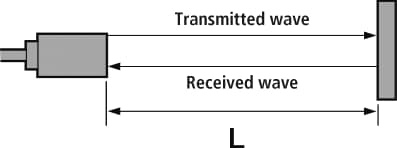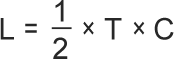# Types of Measurements SystemsUltrasonic Displacement Sensors

## Ultrasonic Method

Ultrasonic displacement sensors emit ultrasonic waves from the sensor head and receive the waves reflected off the target object. The distance is measured by detecting and calculating the time from the emission to receipt of the ultrasonic wave.If the detected distance is expressed as L, the time from the emission of ultrasonic to the receipt as T, and the speed of sound as C, the detected distance can be calculated by the formula: L = 1/2 × T × C. (T is 'to-and-from' time; thus it is divided by 2.)